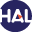# Volume 3 N°2 (2006)

Latest addition : 6 December 2009.

• ### Error estimate for Finite volume approximate solutions of oblique derivative boundary value problem

6 December 2009,

Full Title: Error estimate for Finite volume approximate solutions of some oblique derivative boundary value problems

Abstract:

This paper is an improvment of [BG 05], concerning the Laplace equation with an oblique boundary condition. When the boundary condition involves a regular coefficient, we present a weak formulation of the problem and we prove some existence and uniqueness results of the weak solution. We develop a Finite volume scheme and we prove the convergence of the Finite volume solution to the weak solution, when the mesh size goes to zero. We also present some partial results for the interesting case of a discontinuous coefficient in the boundary condition. In particular, we give a Finite volume scheme, taking in consideration the discontinuities of this coefficient Finally, we obtain some error estimates (in a convenient norm) of order ph (where h is the mesh size), when the solution u is regular enough.

Paper presented by:

Prof. F. BenkhaldounVoir sur HAL : https://hal.archives-ouvertes.fr/hal-01114201

• ### A rough scheme to couple free and porous media

6 December 2009,

Full Title: A rough scheme to couple free and porous media

Abstract:

This paper is devoted to the computation of flows between free and porous media separated by a thin interface . The basic strategy relies on some ideas developed earlier by J.M. Greenberg and A.Y. Leroux on their work on well balanced schemes. This approach requires introducing a set of partial differential equations at the interface, in order to account for the sudden change of medium.

The main features of the interface PDE are investigated. We afterwards propose to compute approximations of solutions with help of an approximate Godunov scheme. A linear interface Riemann solver is introduced, which aims at enforcing the continuity of the two (steady wave-) Riemann invariants. Numerical computations involving shock waves or rarefaction waves are examined and the agreement with the entropy inequality is tracked. Effects of the mesh refinement and the impact of the smoothing of the thin interface are also adressed in the paper.

Paper presented by: Prof. F. BenkhaldounVoir sur HAL : https://hal.archives-ouvertes.fr/hal-01114212

• ### A finite volume approximation for second order elliptic problems

6 December 2009,

Description: Title: A finite volume approximation for second order elliptic problems with a full matrix on quadrilateral grids: derivation of the scheme and a theoretical analysis

Abstract :

We present in this paper a finite-volume based flexible Multi-Point Flux Approximation method (MPFA method, in short) displaying strong capabilities to handle flow problems in non-homogeneous anisotropic media. When the diffusion coefficient governing the flow is a full matrix with constant components, the discrete system is symmetric positive definite even if this matrix is only positive definite. In addition, if the diffusion coefficient is diagonal, the discrete system is reduced to two independent discrete systems corresponding to well-known cell-centered and vertexcentered finite volume methods.

A stability result and error estimates are given in L2 ; and L(infinity);norm and in a discrete energy norm as well. These results have been confirmed by numerical experiments. In this connection, a comparison of our MPFA method with the MPFA O-method has been performed.

Paper presented by:

Prof. F. BenkhaldounVoir sur HAL : https://hal.archives-ouvertes.fr/hal-01120121